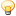CodeGuru Home VC++ / MFC / C++ .NET / C# Visual Basic VB Forums Developer.com

# Thread: 2D line segment handling with data structures

1.Junior MemberJoin Date
Feb 2013
Posts
2

## 2D line segment handling with data structures

I have huge list of 2d line segments. I want to find nearest line segments for a given segment and then to store in a vector. So, I want to do this for each and every line segment. therefore, I know nearest line segments for any line segments at the end (This is what I need). All my line segments are in vector form that is, I know both end point coordinates. So in my data line-number, begin (x,y,z), end(x,y,z) are there.

For obtaining the line segments, I want to check the distance between the line segment and end point of another line segment (this).

I think there might be some data structures that all of these things happen and give a vector of vector line segment numbers or some other way to recognize proximity line segments. I know kd-tree (k-nearest) can do the similar thing. But it is for point data.

I was trying to figure out this with opencv r-tree function. But as I do not have any experience of using r-tree I was not able and I am confused now because it says about a classifier and also something about a training phase. But for my case, I feel I dont want such things to follow.

If anyone knows any function or codes or library to do this thing, please let me know. thanks in advance.Reply With Quote

2. ##Re: 2D line segment handling with data structures

So in my data line-number, begin (x,y,z), end(x,y,z) are there.
If this is a 2D problem, then the points are (x, y). Anyway ...

The shortest distance between a line, defined by points (x1, y1) and (x2, y2), and a third point (x3, y3) is:

Shortest Distance = |(x3 - x1)(y2 - y1) + (x1 - x2)(y3 - y1)| / (Distance between points (x1, y1) and (x2, y2))

For the case of a line segment, the third point could lie in one of three regions. The three regions are separated by two parallel lines which are perpendicular to the line segment. One line passes through (x1, y1) and the other line passes through (x2, y2). If the third point lies in the middle region between these two parallel lines, then the above equation is used to calculate the shortest distance. If the third point lies in the other two regions, then the shortest distance is the distance between the closest endpoint and the third point.Reply With Quote

3.Junior MemberJoin Date
Feb 2013
Posts
2

## Re: 2D line segment handling with data structures

@Coder Dave: thank you for the response. I think I confused you.. (non native english speaker.. sorry). I already done that function. What I am looking is any data structures which can work with line segments. I know r-tree can. But in opencv, all built in r-tree function are developed doing something like classification as classifier, training phases are there. What I am looking for is, any r-tree function which gives proximity line segments (that mean, based on the above distance check function) neigbours should be selected and give a vec of vec line segments.Reply With Quote

algorithms, c++, data structures####Posting Permissions

• You may not post new threads
• You may not post replies
• You may not post attachments
• You may not edit your posts
•